# Drawing primitives batched

OPENRNDR provides special draw APIs for drawing many circles, rectangles or points at once by doing something called “batching”.

This technique performs much faster because the rendering time is tied to the number of calls we do on the GPU. It takes less time to send one large piece of data from CPU to GPU than sending many small pieces. Using this approach can be beneficial when drawing hundreds or thousands of elements.

## Batched circles

This example makes use of `Circle` (a class with properties like `center` and `radius` and some useful methods), not to be confused with `drawer.circle()` (a method that draws pixels on the screen). It is possible to construct a `Circle` by providing a `center` position and a `radius` but also with two or three `Vector2` points that are used for deriving the circumference of a `Circle`.

Calling `drawer.circles()` to draw a list of `Circle` is much faster than calling `drawer.circle()` multiple times.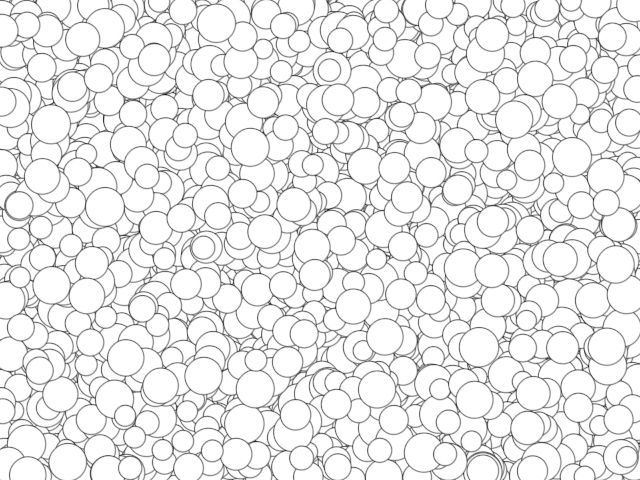``````fun main() = application {
program {
extend {
drawer.clear(ColorRGBa.PINK)
drawer.fill = ColorRGBa.WHITE
drawer.stroke = ColorRGBa.BLACK
drawer.strokeWeight = 1.0

val circles = List(50000) {
Circle(Math.random() * width, Math.random() * height, Math.random() * 10.0 + 10.0)
}
drawer.circles(circles)
}
}
}
``````

`drawer.circles` has several signatures. One of them accepts a list of `Vector2` for the circle centers and a `Double` to specify the radius for all circles. This example draws 5000 circles on the screen leaving a 100.0 pixel margin around the edges.

``````val area = drawer.bounds.offsetEdges(-100.0)
val positions = List(5000) {
Random.point(area)
}
drawer.circles(positions, 20.0)
``````

To have a unique radius per circle we can provide a list of Double as a second argument: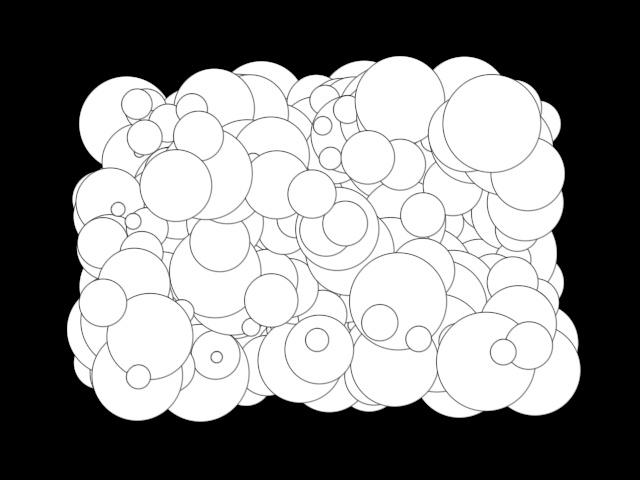``````fun main() = application {
program {
extend {
val area = drawer.bounds.offsetEdges(-100.0)
val positions = List(400) {
Random.point(area)
}
Random.double(5.0, 50.0)
}
}
}
}
``````

What about unique colors and `strokeWeight`s per circle? Creating static or dynamic batches makes it possible, both shown in the next example.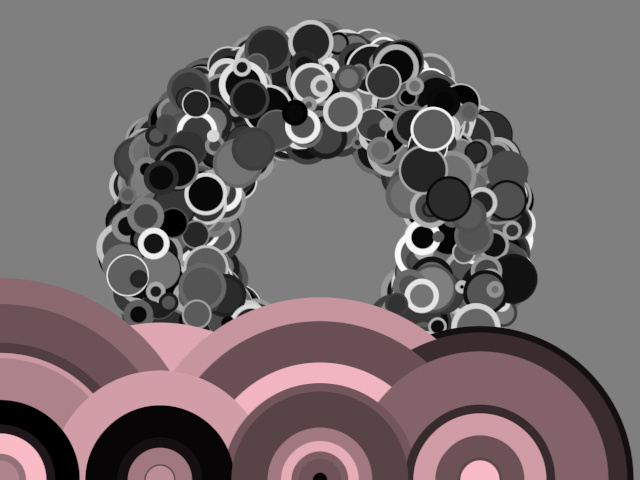``````fun main() = application {
program {
val staticBatch = drawer.circleBatch {
for (i in 0 until 2000) {
strokeWeight = 1 + Math.random() * 5
val pos = Random.ring2d(100.0, 200.0) as Vector2
circle(pos + drawer.bounds.center, 5 + Math.random() * 20)
}
}

extend {
drawer.clear(ColorRGBa.GRAY)
drawer.circles(staticBatch)

// dynamic batch
drawer.circles {
repeat(100) {
stroke = null
val pos = Vector2((it * 160.0) % width, height * 1.0)
val radius = Random.double(2.5, 110.0 - it) * 2
}
}
}
}
}
``````

## Batched rectangles

This example calls the `.rectangle()` method of `RectangleBatchBuilder` multiple times to construct a batch. It does so in two different ways: first, to construct a static batch of monochrome rectangles and second, to construct a dynamic batch of translucent pink rectangles in each animation frame.

The `.rectangle()` method takes two arguments: a `Rectangle` object and an optional rotation. `Rectangle`s can be constructed in different ways: with position, width and optional height or by using `Rectangle.fromCenter()`.

Calling `drawer.rectangles()` to draw a rectangle batch is much faster than calling `drawer.rectangle()` multiple times.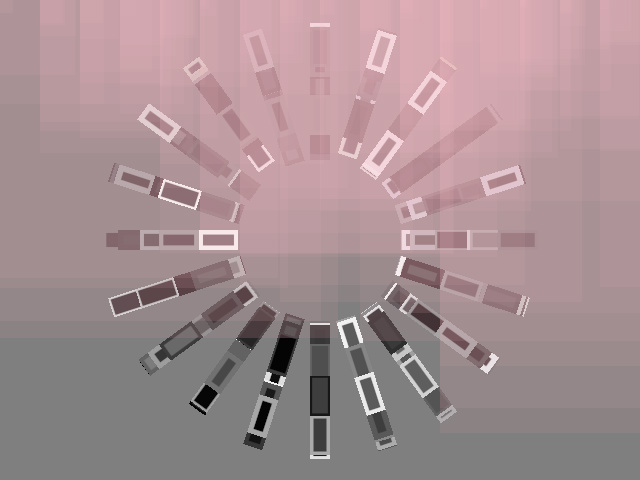``````fun main() = application {
program {
val staticBatch = drawer.rectangleBatch {
for (i in 0 until 1000) {
strokeWeight = Random.double(1.0, 5.0)
val angle = Random.int0(20) * 18.0
val pos = drawer.bounds.center + Polar(angle, Random.double(100.0, 200.0)).cartesian
val rect = Rectangle.fromCenter(pos, width = 40.0, height = 20.0)
rectangle(rect, angle) // add rect to the batch
}
}

extend {
drawer.clear(ColorRGBa.GRAY)
drawer.rectangles(staticBatch)

// dynamic batch
drawer.rectangles {
repeat(100) {
fill = ColorRGBa.PINK.opacify(0.05)
stroke = null
val pos = Vector2((it * 200.0) % width, 0.0)
val size = 5 + Math.random() * Math.random() * height
rectangle(Rectangle(pos, size))
}
}
}
}
}
``````

## Batched points

Drawing batched points is similar to drawing batched circles and rectangles. In this case we use a `PointBatchBuilder` and its `.point()` method. Note that we can specify the color of each point by using `.fill`.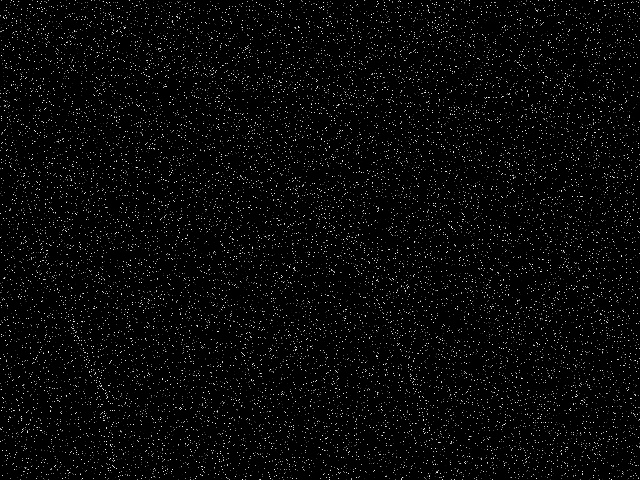``````fun main() = application {
program {
extend {
drawer.clear(ColorRGBa.BLACK)
drawer.points {
repeat(20000) {
fill = rgb((it * 0.01 - seconds) % 1)
point((it * it * 0.011) % width, (it * 4.011) % height)
}
}
}
}
}
``````